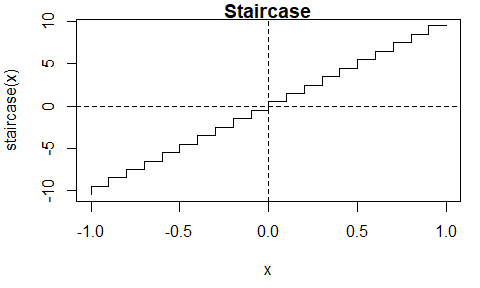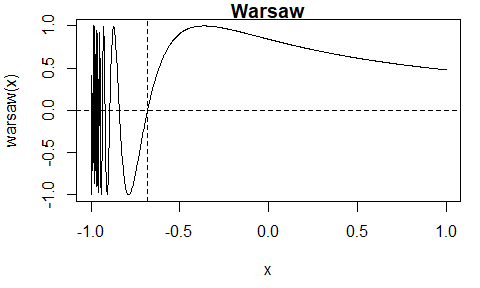## The Interpolate, Truncate, Project (ITP) Root-Finding Algorithm

The itp package implements the Interpolate, Truncate, Project (ITP) root-finding algorithm of Oliveira and Takahashi (2021). Each iteration of the algorithm results in a bracketing interval for the root that is narrower than the previous interval. It’s performance compares favourably with existing methods on both well-behaved functions and ill-behaved functions while retaining the worst-case reliability of the bisection method. For details see the authors’ Kudos summary and the Wikipedia article ITP method.

### Examples

We use three examples from Section 3 of Oliveira and Takahashi (2021) to illustrate the use of the itp function. Each of these functions has a root in the interval . The function can be supplied either as an R function or as an external pointer to a C++ function.

library(itp)

#### A continuous function

The Lambert function is continuous.The itp function finds an estimate of the root, that is, for which is (approximately) equal to 0. The algorithm continues until the length of the interval that brackets the root is smaller than , where is a user-supplied tolerance. The default is .

First, we supply an R function that evaluates the Lambert function.

# Lambert, using an R function
lambert <- function(x) x * exp(x) - 1
itp(lambert, c(-1, 1))
#> function: lambert
#>       root     f(root)  iterations
#>     0.5671   2.048e-12           8

Now, we create an external pointer to a C++ function that has been provided in the itp package and pass this pointer to the function itp(). For more information see the Overview of the itp package vignette.

# Lambert, using an external pointer to a C++ function
lambert_ptr <- xptr_create("lambert")
itp(lambert_ptr, c(-1, 1))
#> function: lambert_ptr
#>       root     f(root)  iterations
#>     0.5671   2.048e-12           8

#### The function itp_c

Also provided is the function itp_c, which is equivalent to itp, but the calculations are performed entirely using C++, and the arguments differ slightly: itp_c has a named required argument pars rather than ... and it does not have the arguments interval, f.a or f.b.

# Calling itp_c()
res <- itp_c(lambert_ptr, pars = list(), a = -1, b = 1)
res
#> function:
#>       root     f(root)  iterations
#>     0.5671   2.048e-12           8

#### A discontinuous function

The staircase function is discontinuous.The itp function finds the discontinuity at at which the sign of the function changes. The value of 0.5 returned for the root res$root is the midpoint of the bracketing interval [res$a, res\$b] at convergence.

# Staircase
staircase <- function(x) ceiling(10 * x - 1) + 1 / 2
res <- itp(staircase, c(-1, 1))
print(res, all = TRUE)
#> function: staircase
#>       root     f(root)  iterations           a           b         f.a
#>  7.404e-11         0.5          31           0   1.481e-10        -0.5
#>        f.b   precision
#>        0.5   7.404e-11

#### A function with multiple roots

The Warsaw function has multiple roots.When the initial interval is the itp function finds the root . There are other roots that could be found from a different initial interval.

# Warsaw
warsaw <- function(x) ifelse(x > -1, sin(1 / (x + 1)), -1)
itp(warsaw, c(-1, 1))
#> function: warsaw
#>       root     f(root)  iterations
#>    -0.6817  -5.472e-11          11

### Installation

To get the current released version from CRAN:

install.packages("itp")

### Vignette

See the Overview of the itp package vignette, which can also be accessed using vignette("itp-vignette", package = "itp").